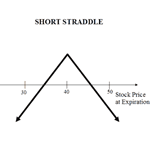April 18, 2012A Short Straddle is a neutral options strategy which produces a profit when the underlying stock doesn’t move much up or down.  It’s essentially the opposite of a Long Straddle which profits when the underlying stock makes a big move up or down.

So, if you think your stock is going to move sideways for a while, you could use a Short Straddle as a way to generate some profits.

How do you establish a short straddle position?

All you have to do is sell both a call option and a put option on the same underlying stock.  The key is to make sure both options have the same strike price and expiration date.

In other words, you’re establishing both a short call option and a short put option on the same stock.  The short call option allows you to profit if the underlying stock moves sideways or down.  The short put option makes you money if the underlying stock moves sideways or up.

When you combine the two short options into a Short Straddle, you have an options strategy than can profit whether the underlying stock remains stagnant or stays within a tight price range.

However, you must use caution with this strategy as your maximum potential loss is unlimited.

Let’s take a look at the following example for an illustration of how a Short Straddle position would work.

Let’s say you want to establish a short straddle on General Electric (GE), which is currently trading at \$20 per share.  You would do this by simultaneously selling one GE Jan \$20 call and one GE Jan \$20 put.  Let’s assume the call option premium is \$3.00 and the put option premium is \$3.30.

The maximum profit you can earn on this position is equal to the net credit received.  In other words, your maximum potential profit is equal to the sum of the two premiums received (\$3.00 + \$3.30) or \$6.30.

This occurs when both of your short call and short put options expire worthless.  They will both expire worthless when the underlying stock closes exactly at the strike price on the expiration date.

To give you an idea of just how well a short straddle could perform, you can calculate your potential percentage return.  This is done using the following formula:

% Return = net credit / [(strike price + highest option bid) – net credit]

Using the figures from our example…

% Return = \$6.30 / [(\$20.00 + \$3.30) – \$6.30)] = \$6.30 / \$17.00 = 37%

So, in our example, the highest percentage return we can make is 37%.  Not too shabby.

Just like with other option strategies, you’re going to want to calculate your breakeven points for the trade.  As with other straddle position, you have two breakeven points, an upper and a lower.  Here’s how you calculate the two breakeven points for a short straddle.

Upper Breakeven = strike price + net credit

Lower Breakeven = strike price – net credit

Applying the figures from our example you end up with:

Upper Breakeven = \$20.00 + \$6.30 = \$26.30

Lower Breakeven = \$20.00 – \$6.30 = \$13.70